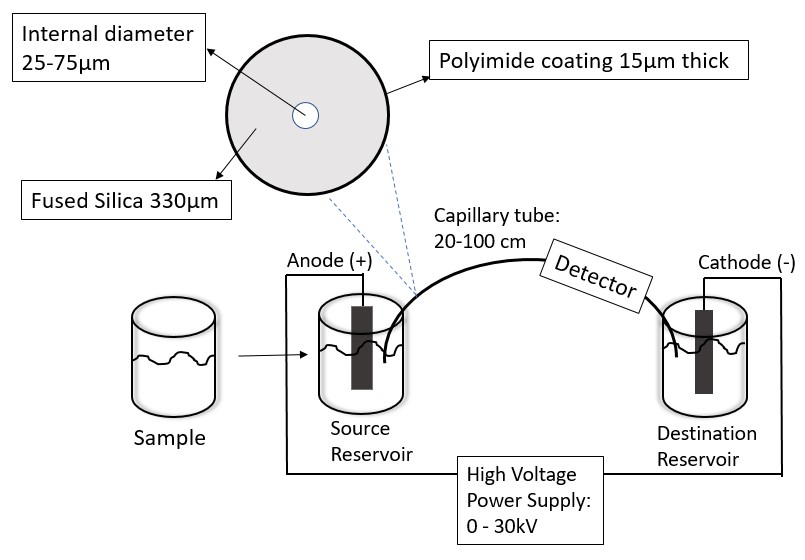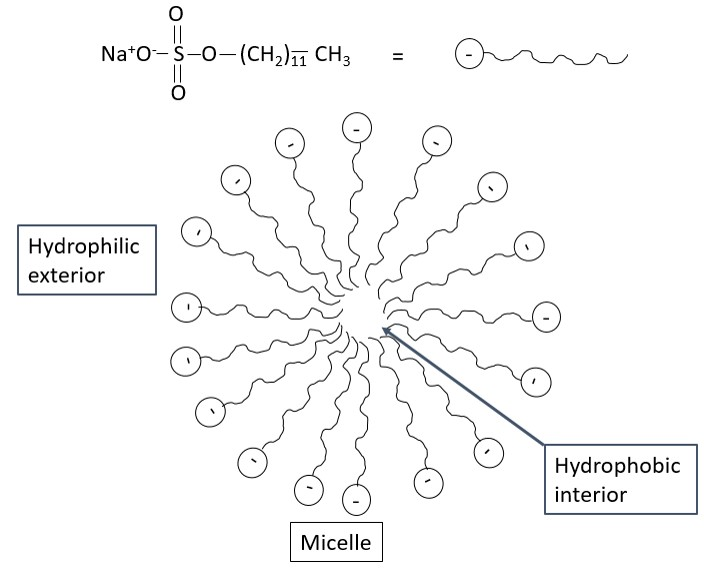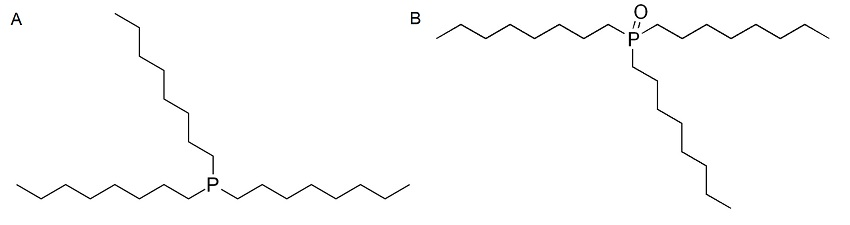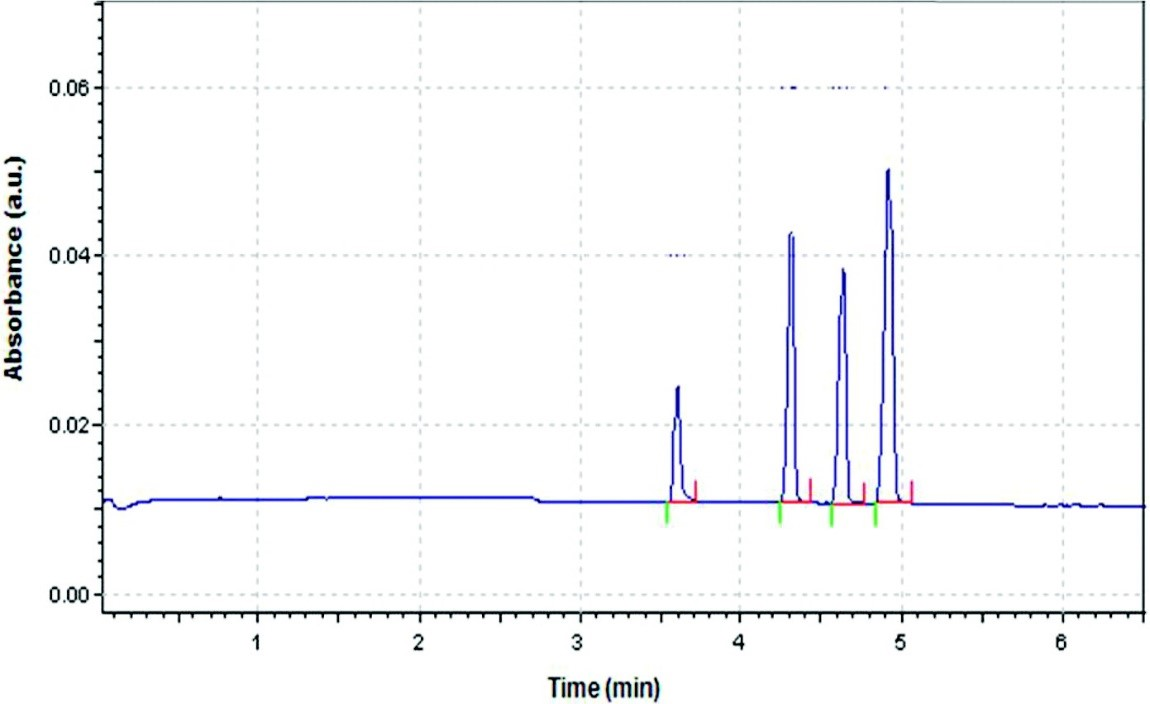# 3.6: Capillary Electrophoresis

$$\newcommand{\vecs}{\overset { \rightharpoonup} {\mathbf{#1}} }$$ $$\newcommand{\vecd}{\overset{-\!-\!\rightharpoonup}{\vphantom{a}\smash {#1}}}$$$$\newcommand{\id}{\mathrm{id}}$$ $$\newcommand{\Span}{\mathrm{span}}$$ $$\newcommand{\kernel}{\mathrm{null}\,}$$ $$\newcommand{\range}{\mathrm{range}\,}$$ $$\newcommand{\RealPart}{\mathrm{Re}}$$ $$\newcommand{\ImaginaryPart}{\mathrm{Im}}$$ $$\newcommand{\Argument}{\mathrm{Arg}}$$ $$\newcommand{\norm}{\| #1 \|}$$ $$\newcommand{\inner}{\langle #1, #2 \rangle}$$ $$\newcommand{\Span}{\mathrm{span}}$$ $$\newcommand{\id}{\mathrm{id}}$$ $$\newcommand{\Span}{\mathrm{span}}$$ $$\newcommand{\kernel}{\mathrm{null}\,}$$ $$\newcommand{\range}{\mathrm{range}\,}$$ $$\newcommand{\RealPart}{\mathrm{Re}}$$ $$\newcommand{\ImaginaryPart}{\mathrm{Im}}$$ $$\newcommand{\Argument}{\mathrm{Arg}}$$ $$\newcommand{\norm}{\| #1 \|}$$ $$\newcommand{\inner}{\langle #1, #2 \rangle}$$ $$\newcommand{\Span}{\mathrm{span}}$$$$\newcommand{\AA}{\unicode[.8,0]{x212B}}$$

Capillary electrophoresis (CE) encompasses a family of electrokinetic separation techniques that uses an applied electric field to separate out analytes based on their charge and size. The basic principle is hinged upon that of electrophoresis, which is the motion of particles relative to a fluid (electrolyte) under the influence of an electric field. The founding father of electrophoresis, Arne W. K. Tiselius (Figure $$\PageIndex{1} a$$ ), first used electrophoresis to separate proteins, and he went on to win a Nobel Prize in Chemistry in 1948 for his work on both electrophoresis and adsorption analysis. However, it was Stellan Hjerten (Figure $$\PageIndex{1} b$$ ) who worked under Arne W. K. Tiselius, who pioneered work in CE in 1967, although CE was not well recognized until 1980 when James W. Jorgenson (Figure $$\PageIndex{1} c$$ ) and Krynn D. Lukacs published a series of papers describing this new technique.Figure $$\PageIndex{1}$$: (left) Swedish chemist Arne W. K. Tiselius (1902–1971) who was the founding father of electrophoresis. (center) Swedish chemist Stellan Hjerten (1928–present) who worked under Arne W. K. Tiselius that pioneered work in CE. (right) James W. Jorgensen (1952-present).

### Instrument Overview

The main components of CE are shown in Figure $$\PageIndex{2}$$. The electric circuit of the CE is the heart of the instrument.Figure $$\PageIndex{2}$$ A schematic diagram of the components of a typical capillary electrophoresis setup and the capillary column.

#### Injection Methods

The samples that are studied in CE are mainly liquid samples. A typical capillary column has an inner diameter of 50 μm and a length of 25 cm. Because the column can only contain a minimal amount of running buffer, only small sample volumes can be tested (nL to μL). The samples are introduced mainly by two injection methods: hydrodynamic and electrokinetic injection. The two methods are displayed in Table $$\PageIndex{1}$$ A disadvantage of electrokinetic injection is that the composition of the injected sample may not be the same as the composition of the original sample. This is because the injection method is dependent on the electrophoretic and electroosmotic mobility of the species in the sample. However, both injection methods depend on the temperature and the viscosity of the solution. Hence, it is important to control both parameters when a reproducible volume of sample injections is desired. It is advisable to use internal standards instead of external standards when performing quantitative analysis on the samples as it is hard to control both the temperature and viscosity of the solution.

Injection Methods Working Principle
Hydrodynamic Injection The sample vial is enclosed in a chamber with one end of fixed capillary column immersed in it. Pressure is then applied to the chamber for a fixed period so that the sample can enter the capillary. After the sample, has been introduced, the capillary is withdrawn and then re-immersed into the source reservoir and separation takes place.
Electrokinetic Injection The sample is enclosed in a chamber with one end of capillary column immersed in it with an electrode present. The electric field is applied, and the samples enter the capillary. After the sample, has been introduced, the capillary is withdrawn and then re-immersed into the source reservoir and separation takes place.
Table $$\PageIndex{1}$$ The working principle of the two injection methods used in CE.

## Column

After the samples have been injected, the capillary column is used as the main medium to separate the components. The capillary column used in CE shares the same characteristics as the capillary column used in gas chromatography (GC); however, the most critical components of the CE column are:

• the inner diameter of the capillary,
• the total length of the capillary,
• the length of the column from the injector to the detector.

#### Solvent Buffer

The solvent buffer carries the sample through the column. It is crucial to employ a good buffer as a successful CE experiment is hinged upon this. CE is based on the separation of charges in an electric field. Therefore, the buffer should either sustain the pre-existing charge on the analyte or enable the analyte to obtain a charge, and it is important to consider the pH of the buffer before using it.

#### Applied Voltage (kV)

The applied voltage is important in the separation of the analytes as it drives the movement of the analyte. It is important that it is not too high as it may become a safety concern.

#### Detectors

Analytes that have been separated after the applying the voltage can be detected by many detection methods. The most common method is UV-visible absorbance. The detection takes place across the capillary with a small portion of the capillary acting as the detection cell. The on-tube detection cell is usually made optically transparent by scraping off the polyimide coating and coating it with another optically transparent material so that the capillary would not break easily. For species that do not have a chromophore, a chromophore can be added to the buffer solution. When the analyte passes by, there would be a decrease in signal. This decreased signal will correspond to the amount of analyte present. Other common detection techniques employable in CE are fluorescence and mass spectrometry (MS).

## Theory

In CE, the sample is introduced into the capillary by the above-mentioned methods. A high voltage is then applied causing the ions of the sample to migrate towards the electrode in the destination reservoir, in this case, the cathode. Sample components migration and separation are determined by two factors, electrophoretic mobility and electroosmotic mobility.

#### Electrophoretic Mobility

The electrophoretic mobility, $$μ_{ep}$$, is inherently dependent on the properties of the solute and the medium in which the solute is moving. Essentially, it is a constant value, that can be calculated as given by \ref{1} where $$q$$ is the solutes charge, $$η$$ is the buffer viscosity and $$r$$ is the solute radius.

$\mu _{ep} = \dfrac{q}{6\pi \eta r} \label{1}$

The electrophoretic velocity, $$v_{ep}$$, is dependent on the electrophoretic mobility and the applied electric field, $$E$$ (\ref{2}).

$\nu _{ep} = \mu _{ep} E \label{2}$

Thus, when solutes have a larger charge to size ratio the electrophoretic mobility and velocity will increase. Cations and the anion would move in opposing directions corresponding to the sign of the electrophoretic mobility with is a result of their charge. Thus, neutral species that have no charge do not have an electrophoretic mobility.

#### Electroosmotic Mobility

The second factor that controls the migration of the solute is the electroosmotic flow. With zero charge, it is expected that the neutral species should remain stationary. However, under normal conditions, the buffer solution moves towards the cathode as well. The cause of the electroosmotic flow is the electric double layer that develops at the silica solution interface.

At pH more than 3, the abundant silanol (-OH) groups present on the inner surface of the silica capillary, de-protonate to form negatively charged silanate ions (-SiO-). The cations present in the buffer solution will be attracted to the silanate ions and some of them will bind strongly to it forming a fixed layer. The formation of the fixed layer only partially neutralizes the negative charge on the capillary walls. Hence, more cations than anions will be present in the layer adjacent to the fixed layer, forming the diffuse layer. The combination of the fixed layer and diffuse layer is known as the double layer as shown in Figure $$\PageIndex{3}$$. The cations present in the diffuse layer will migrate towards the cathode, as these cations are solvated the solution will also flow with it, producing the electroosmotic flow. The anions present in the diffuse layer are solvated and will move towards the anode. However, as there are more cations than anions the cations will push the anions together with it in the direction of the cathode. Hence, the electroosmotic flow moves in the direction of the cathode.Figure $$\PageIndex{3}$$: An illustration of the electric double layer and movement of the species in solution. Adapted from D. Harvey, Analytical Chemistry 2.0(e-textbook), 851.

The electroosmotic mobility, μeof, is described by \ref{3} where ξ is the zeta potential, ε is the buffer dielectric constant and η is the buffer viscosity. The electroosmotic velocity, veof, is the rate at which the buffer moves through the capillary is given by \ref{4} .

$\mu _{eof} \ =\ \frac{\zeta \varepsilon }{4\pi \eta } \label{3}$

$\nu _{eof}\ =\ \mu _{eof}E \label{4}$

#### Zeta Potential

The zeta potential, ξ, also known as the electrokinetic potential is the electric potential at the interface of the double layer. Hence, in our case, it is the potential of the diffuse layer that is at a finite distance from the capillary wall. Zeta potential is mainly affected and directly proportional to two factors:

1. The thickness of the double layer. A higher concentration of cations possibly due to an increase in the buffers ionic strength would lead to a decrease in the thickness of the double layer. As the thickness of the double layer decreases, the zeta potential would decrease that results in the decrease of the electroosmotic flow.
2. The charge on the capillary walls. A greater density of the silanate ions corresponds to a larger zeta potential. The formation of silanate ions is pH dependent. Hence, at pH less than 2 there is a decrease in the zeta potential and the electroosmotic flow as the silanol exists in its protonated form. However, as the pH increases, there are more silanate ions formed causing an increase in zeta potential and hence, the electroosmotic flow.Figure $$\PageIndex{4}$$ An illustration of the order of elution of the charged species. Adapted from D. A. Skoog, D. M. West, F. J. Holler and S. R. Crouch, Fundamentals of Analytical Chemistry, Copyright Brooks Cole (2013).

#### Order of Elution

Electroosmotic flow of the buffer is generally greater than the electrophoretic flow of the analytes. Hence, even the anions would move to the cathode as illustrated in Figure $$\PageIndex{4}$$. Small, highly charged cations would be the first to elute before larger cations with lower charge. This is followed by the neutral species which elutes as one band in the middle. The larger anions with low charge elute next and lastly, the highly charged small anion would have the longest elution time. This is clearly portrayed in the electropherogram in Figure $$\PageIndex{5}$$.Figure $$\PageIndex{5}$$ A typical electropherogram demonstrating the order of elution of cations and anions. Adapted from J. Sáiz, I. J. Koenka, T. Duc Mai, P. C. Hauser, C. García-Ruiz, TrAC, 2014, 62. 162.

### Optimizing the CE Experiment

There are several components that can be varied to optimize the electropherogram obtained from CE. Hence, for any given setup certain parameters should be known:

• the total length of the capillary (L),
• the length the solutes travel from the start to the detector (l),
• the applied voltage (V).

#### Reduction in Migration Time, tmn

To shorten the analysis time, a higher voltage can be used or a shorter capillary tube can be used. However, it is important to note that the voltage cannot be arbitrarily high as it will lead to joule heating. Another possibility is to increase μeof by increasing pH or decreasing the ionic strength of the buffer, \ref{5} .

$t_{mn} \ =\ \frac{1\ L}{(\mu _{ep} \ +\ \mu_{eof}) V } \label{5}$

#### Efficiency

In chromatography, the efficiency is given by the number of theoretical plates, N. In CE, there exist a similar parameter, \ref{6} where D is the solute`s diffusion coefficient. Efficiency increase s with an increase in voltage applied as the solute spends less time in the capillary there will be less time for the solute to diffuse. Generally, for CE, N will be very large.

$N\ =\frac{1^{2}}{2Dt_{mn}} = \frac{\mu _{tot} V l}{2DL} \label{6}$

#### Resolution Between Two Peaks

The resolution between two peaks, R, is defined by \ref{7} where Δv is the difference in velocity of two solutes and ṽ is the average velocity of two solutes.

$R= \frac{\sqrt{N} }{4} \times \frac{\Delta v}{ \tilde{\nu } } \label{7}$

Substituting the equation by N gives \ref{8}

$R\ = 0.177(\mu _{ep,1} \ -\ \mu _{ep,2}) \sqrt{ \frac{V}{D(\nu _{av} + \mu _{eof})} } \label{8}$

Therefore, increasing the applied voltage, V, will increase the resolution. However, it is not very effective as a 4-fold increase in applied voltage would only give a 2-fold increase in resolution. In addition, increase in N, the number of theoretical plates would result in better resolution.

#### Selectivity

In chromatography, selectivity, α, is defined as the ratio of the two retention factors of the solute. This is the same for CE, \ref{9} , where t2 and t1 are the retention times for the two solutes such that, α is more than 1.

$\alpha =\frac{t_{2}}{t_{1}} \label{9}$

Selectivity can be improved by adjusting the pH of the buffer solution. The purpose is to change the charge of the species being eluted.

### Comparison Between CE and HPLC

CE unlike High-performance liquid chromatography (HPLC) accommodates many samples and tends to have a better resolution and efficiency. A comparison between the two methods is given in Table $$\PageIndex{2}$$.

CE HPLC
Wider selection of analyte to be analyzed Limited by the solubility of the sample
Higher efficiency, no stationary mass transfer term as there is no stationary phase Efficiency is lowered due to the stationary mass transfer term (equilibration between the stationary and mobile phase)
Electroosmotic flow profile in the capillary is flat as a result no band broadening. Better peak resolution and sharper peaks Rounded laminar flow profile that is common in pressure driven systems such as HPLC. Resulting in broader peaks and lower resolution
Can be coupled to most detectors depending on application Some detectors require the solvent to be changed and prior modification of the sample before analysis
Greater peak capacity as it uses a very large number of theoretical plates, N The peak capacity is lowered as N is not as large
High voltages are used when carrying out the experiment No need for high voltage
Table $$\PageIndex{2}$$ Advantages and disadvantages of CE versus HPLC.

### Micellar Electrokinetic Chromatography

CE allows the separation of charged particles, and it is mainly compared to ion chromatography. However, no separation takes place for neutral species in CE. Thus, a modified CE technique named micellar electrokinetic chromatography (MEKC) can be used to separate neutrals based on its size and its affinity to the micelle. In MEKC, surfactant species is added to the buffer solution at a concentration at which micelles will form. An example of a surfactant is sodium dodecyl sulfate (SDS) as seen in Figure $$\PageIndex{6}$$Figure $$\PageIndex{6}$$: The structure of sodium dodecyl sulfate and its representation. An illustration of a cross section of a formed micelle. Adapted from D. Harvey, Analytical Chemistry 2.0(e-textbook), 851.

Neutral molecules are in dynamic equilibrium between the bulk solution and interior of the micelle. In the absence of the micelle the neutral species would reach the detector at t0 but in the presence of the micelle, it reaches the detector at tmc, where tmc is greater than t0. The longer the neutral molecule remains in the micelle, the longer it's migration time. Thus small, non-polar neutral species that favor interaction with the interior of the micelle would take a longer time to reach the detector than a large, polar species. Anionic, cationic and zwitter ionic surfactants can be added to change the partition coefficient of the neutral species. Cationic surfactants would result in positive micelles that would move in the direction of electroosmotic flow. This enables it to move faster towards the cathode. However, due to the fast migration, it is possible that insufficient time is given for the neutral species to interact with the micelle resulting in poor separation. Thus, all factors must be considered before choosing the right surfactant to be used. The mechanism of separation between MEKC and liquid chromatography is the same. Both are dependent on the partition coefficient of the species between the mobile phase and stationary phase. The main difference lies in the pseudo stationary phase in MEKC, the micelles. The micelle which can be considered the stationary phase in MEKC moves at a slower rate than the mobile ions.

##### Case Study: The Use of CE in Separation of Quantum Dots

Quantum dots (QD) are semiconductor nanocrystals that lie in the size range of 1-10 nm, and they have different electrophoretic mobility due to their varying sizes and surface charge. CE can be used to separate and characterize such species, and a method to characterize and separate CdSe QD in the aqueous medium has been developed. The QDs were synthesized with an outer layer of trioctylphosphine (TOP, Figure $$\PageIndex{7} a$$) and trioctylphosphine oxide (TOPO, Figure $$\PageIndex{7} b$$), making the surface of the QD hydrophobic. The background electrolyte solution used was SDS, in order to make the QDs soluble in water and form a QD-TOPO/TOP-SDS complex. Different sizes of CdSe were used and the separation was with respect to the charge-to-mass ratio of the complexes. It was concluded from the study that the larger the CdSe core (i.e., the larger the charge-to-mass ratio) eluted out last. The electropherogram from the study is shown in Figure $$\PageIndex{8}$$ from which it is visible that good separation had taken place by using CE. Laser-induced fluorescence detection was used, the buffer system was SDS, and the pH of the system set up was fixed at 6.5. The pH is highly important in this case as the stability of the system and the separation is dependent on it.Figure $$\PageIndex{7}$$ A. The structure of trioctylphosphine (TOP). B. The structure of trioctylphosphine oxide (TOPO).Figure $$\PageIndex{8}$$ Electropherogram for a mixture of four different CdSe-TOPO/TOP-SDS complexes. Reproduced from C. Carrillo-Carrión, Y. Moliner-Martínez, B. M. Simonet, and M. Valcárcel, Anal. Chem., 2011, 83, 2807

This page titled 3.6: Capillary Electrophoresis is shared under a CC BY 4.0 license and was authored, remixed, and/or curated by Pavan M. V. Raja & Andrew R. Barron (OpenStax CNX) via source content that was edited to the style and standards of the LibreTexts platform; a detailed edit history is available upon request.